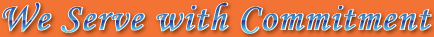### Dimension Weight

Calculate the dimensional weight of your shipment

Sometimes, large items with a light overall weight can be charged according to the space they take up on aircraft. In these cases, Dimensional Weight (Dim) is used to calculate the shipment cost.

It is recommended that you calculate the Dimensional Weight for every shipment that you send, then compare this to its actual weight. The greater weight of the two is used to work out the price that we charge you.

International Dimensional Weights are calculated using the formula below:
Length x Width x Height in centimeters / 6000 = Dimensional Weight in kilograms

Please feel free to use our quick calculator below.Dimensional Weight

KG

Length

cm

Weight

cm

Height

cm